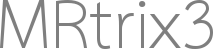# 5ttgen with HCP data

Dear expert,

I am running the command:

`5ttgen fsl T1w_acpc_dc_restore_brain.nii.gz 5TT.mif -premasked`

for some subject everything went smoothly but for some of them I got an empty image. I’ve seen @rsmith post on processing HCP data (Processing of HCP data), so I know that the segmentation with this dataset is an issue.

I was wondering whether if you have solved it by any chance? Would you have any suggestion?

Thanks

Hi Davide,

It’s difficult to provide any feedback based on the limited feedback of having produced an empty image. The first step would be to re-run the script with the `-nocleanup` option, go into the scratch directory, then step through the sequence of commands listed in file `log.txt` until you find the first image / file for which the contents are not what would be expected. That would isolate which specific component of the script’s logic is responsible for the erroneous output.

Cheers
Rob

Hi @rsmith,

thanks for your suggestions. Indeed, I did it but I was not able to figure out what it is going on with these files given that for mostly of the HCP subjects everything works fine.

I’ve uploaded one of those file here:

Could you please check it out? It would be highly appreciateThanks anyway

Hi Davide,

The error is occurring within FSL `fast`, and is therefore largely out of my control. You could try experimenting with the FAST command-line parameters to see what mitigates the issue; if you find something that works, then with a bit of manual manipulation you could use the `-continue` option to execute the rest of the script.

FAST terminal output (click to expand)
``````\$ fast -v T1.nii
Starting Single Image Segmentation
T1-weighted image
Imagesize : 256 x 256 x 256
Pixelsize : 1 x 1 x 1

1 4.14313
2 4.41884
3 4.66344
KMeans Iteration 0
KMeans Iteration 1
KMeans Iteration 2
KMeans Iteration 3
KMeans Iteration 4
KMeans Iteration 5
KMeans Iteration 6
KMeans Iteration 7
KMeans Iteration 8
KMeans Iteration 9
KMeans Iteration 10
KMeans Iteration 11
KMeans Iteration 12
KMeans Iteration 13
KMeans Iteration 14
KMeans Iteration 15
KMeans Iteration 16
KMeans Iteration 17
KMeans Iteration 18
Tanaka Iteration 0 bias field 4
Tanaka-inner-loop-iteration=0 MRFWeightsTotal=1.41742e+07 beta=0.02
Tanaka-inner-loop-iteration=1 MRFWeightsTotal=1.43116e+07 beta=0.02
Tanaka-inner-loop-iteration=2 MRFWeightsTotal=1.43147e+07 beta=0.02
Tanaka-inner-loop-iteration=3 MRFWeightsTotal=1.43147e+07 beta=0.02
Tanaka-inner-loop-iteration=4 MRFWeightsTotal=1.43147e+07 beta=0.02
CLASS 1 MEAN 29.7523 STDDEV 35.6565 CLASS 2 MEAN 83.6975 STDDEV 13.2416 CLASS 3 MEAN 110.915 STDDEV 0.669781
Tanaka Iteration 1 bias field 4
Tanaka-inner-loop-iteration=0 MRFWeightsTotal=1.42336e+07 beta=0.02
Tanaka-inner-loop-iteration=1 MRFWeightsTotal=1.43709e+07 beta=0.02
Tanaka-inner-loop-iteration=2 MRFWeightsTotal=1.4374e+07 beta=0.02
Tanaka-inner-loop-iteration=3 MRFWeightsTotal=1.43741e+07 beta=0.02
Tanaka-inner-loop-iteration=4 MRFWeightsTotal=1.43741e+07 beta=0.02
CLASS 1 MEAN 28.9411 STDDEV 34.5821 CLASS 2 MEAN 83.9975 STDDEV 13.3864 CLASS 3 MEAN 110.89 STDDEV 0.517345
Tanaka Iteration 2 bias field 4
Tanaka-inner-loop-iteration=0 MRFWeightsTotal=1.42606e+07 beta=0.02
Tanaka-inner-loop-iteration=1 MRFWeightsTotal=1.43994e+07 beta=0.02
Tanaka-inner-loop-iteration=2 MRFWeightsTotal=1.44026e+07 beta=0.02
Tanaka-inner-loop-iteration=3 MRFWeightsTotal=1.44027e+07 beta=0.02
Tanaka-inner-loop-iteration=4 MRFWeightsTotal=1.44027e+07 beta=0.02
CLASS 1 MEAN 28.9273 STDDEV 34.5889 CLASS 2 MEAN 84.3536 STDDEV 13.6325 CLASS 3 MEAN 110.938 STDDEV 0.362045
Tanaka Iteration 3 bias field 4
Tanaka-inner-loop-iteration=0 MRFWeightsTotal=1.43417e+07 beta=0.02
Tanaka-inner-loop-iteration=1 MRFWeightsTotal=1.44857e+07 beta=0.02
Tanaka-inner-loop-iteration=2 MRFWeightsTotal=1.44891e+07 beta=0.02
Tanaka-inner-loop-iteration=3 MRFWeightsTotal=1.44892e+07 beta=0.02
Tanaka-inner-loop-iteration=4 MRFWeightsTotal=1.44892e+07 beta=0.02
CLASS 1 MEAN 28.9618 STDDEV 34.6203 CLASS 2 MEAN 84.5622 STDDEV 13.7749 CLASS 3 MEAN 110.96 STDDEV 0.240898
Tanaka Iteration 0 hyperparameter 4
Tanaka-inner-loop-iteration=0 MRFWeightsTotal=0 beta=0.02
Tanaka-inner-loop-iteration=1 MRFWeightsTotal=0 beta=0.02
Tanaka-inner-loop-iteration=2 MRFWeightsTotal=0 beta=0.02
Tanaka-inner-loop-iteration=3 MRFWeightsTotal=0 beta=0.02
Tanaka-inner-loop-iteration=4 MRFWeightsTotal=0 beta=0.02
CLASS 1 MEAN 29.2546 STDDEV 35.082 CLASS 2 MEAN 85.0898 STDDEV 14.1288 CLASS 3 MEAN 110.99 STDDEV -nan
BETA 0.1
Tanaka Iteration 1 hyperparameter 4
Tanaka-inner-loop-iteration=0 MRFWeightsTotal=0 beta=0.1
Tanaka-inner-loop-iteration=1 MRFWeightsTotal=0 beta=0.1
Tanaka-inner-loop-iteration=2 MRFWeightsTotal=0 beta=0.1
Tanaka-inner-loop-iteration=3 MRFWeightsTotal=0 beta=0.1
Tanaka-inner-loop-iteration=4 MRFWeightsTotal=0 beta=0.1
CLASS 1 MEAN -nan STDDEV -nan CLASS 2 MEAN -nan STDDEV -nan CLASS 3 MEAN -nan STDDEV -nan
BETA 0.1
Tanaka Iteration 2 hyperparameter 4
Tanaka-inner-loop-iteration=0 MRFWeightsTotal=0 beta=0.1
Tanaka-inner-loop-iteration=1 MRFWeightsTotal=0 beta=0.1
Tanaka-inner-loop-iteration=2 MRFWeightsTotal=0 beta=0.1
Tanaka-inner-loop-iteration=3 MRFWeightsTotal=0 beta=0.1
Tanaka-inner-loop-iteration=4 MRFWeightsTotal=0 beta=0.1
CLASS 1 MEAN -nan STDDEV -nan CLASS 2 MEAN -nan STDDEV -nan CLASS 3 MEAN -nan STDDEV -nan
BETA 0.1
Tanaka Iteration 3 hyperparameter 4
Tanaka-inner-loop-iteration=0 MRFWeightsTotal=0 beta=0.1
Tanaka-inner-loop-iteration=1 MRFWeightsTotal=0 beta=0.1
Tanaka-inner-loop-iteration=2 MRFWeightsTotal=0 beta=0.1
Tanaka-inner-loop-iteration=3 MRFWeightsTotal=0 beta=0.1
Tanaka-inner-loop-iteration=4 MRFWeightsTotal=0 beta=0.1
CLASS 1 MEAN -nan STDDEV -nan CLASS 2 MEAN -nan STDDEV -nan CLASS 3 MEAN -nan STDDEV -nan
BETA 0.1
Starting Partial Volume Estimation

tissue 1 1.33222e+06
tissue 2 0
tissue 3 0
total tissue 1.33222e+06
``````

Cheers
Rob

Thanks @rsmith,

in the end I use a Gaussian Mixture Models (GMM) to obtain a segmentation and then build the 5tt image myself.
Only a quick question: does it change something if for mostly of the subjects I use fast segmentation algorithm and for few of them the GMM? The segmentation looks pretty good to me

Thanks

in the end I use a Gaussian Mixture Models (GMM) to obtain a segmentation and then build the 5tt image myself.

If this is something that you find useful, and the relevant processing is available at the command line, you could write a new `5ttgen` algorithm wrapping that functionalitydoes it change something if for mostly of the subjects I use fast segmentation algorithm and for few of them the GMM?

Depends entirely on your downstream analysis. As a generic argument, performing different processing for different subjects always has the potential to be a problem when trying to make statistical inferences. You could at least include this variable as a nuisance regressor. Conversely, if all you’re doing is generating and visualising streamlines, then it doesn’t really matter at all. So it depends on where within that spectrum your intentions lie.